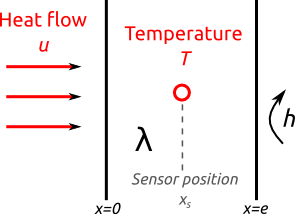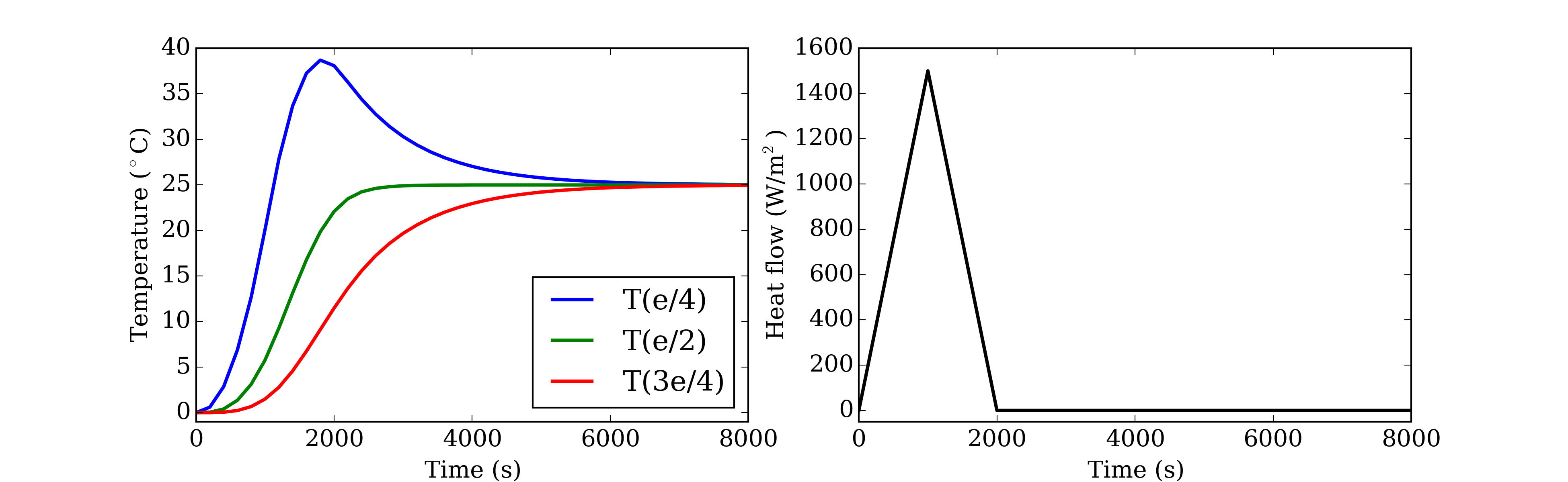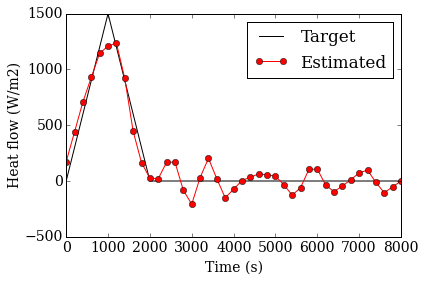# 02_HeatFlow¶

## Linear inverse problem: identifying the heat flow in a wall from transient temperature measurements¶

This page is a tutorial on how to solve the inverse heat conduction problem (IHCP) with Python code. It is mostly based on this series of articles (in French) that give a great introduction to inverse problems in heat transfer.

## 1. Introduction¶

The physical problem is 1D transient heat flow in a finite wall:

In :
%matplotlib inline
from IPython.display import Image
Image(filename='figures/IHCP.png', width = 240)

Out:In the previous tutorial, we used the same layout to assess the thermal conductivity $\lambda$ and the sensor position, assuming that measurements of the boundary heat flow and of the temperature inside the wall were available (with some measurement uncertainty, which we evaluated as well).

The present exercise is more problematic: this time, we want to identify a time-varying quantity $u(t)$, rather than fixed material properties. However, there is good news: this problem involves a white-box linear model, and may be solved in a more direct way.

In :
Image(filename='figures/input_output.png', width = 800)

Out:The input to the inverse problem are measurements of temperature, which may have been recorded anywhere within the wall (see left panel of the figure above). The target is to reconstruct the history of the heat flow on the left boundary of the wall (see right panel of the figure above). This time, the thermal conductivity $\lambda$ and sensor position $x_s$ are known.

Since the heat flow is a function of time, the trick to make it identifiable by the inverse problem is to parameterise it into a finite set of $n$ elementary functions $f_j$: \begin{equation} u\left(t\right) = \sum_{j=1}^{n}{u_j f_j(t)} \end{equation} Hence, the problem actually has $n$ unknowns, assembled into a vector u = [$u_j; j \in \{1...n\}$]. The proper choice for the number of elementary functions is not really the topic of this tutorial, although it is an important issue.

## 2. Background¶

### Model¶

The physical model is the 1D heat conduction equation in a finite medium of heat capacity $\rho c_p$ and conductivity $\lambda$:

\begin{align} \rho c_p \frac{\partial T}{\partial t} = \lambda \frac{ \partial^2 T}{\partial x^2} \end{align}

Assorted with the following boundary conditions: a prescribed heat flow $u(t)$ on the left boundary and a type-3 condition ($h$, $T_\infty$) on the right boundary

\begin{align} -\lambda \dfrac{\partial T}{\partial x} & = u\left(t\right) & \quad \mathrm{if} \quad x = 0 \\ -\lambda \dfrac{\partial T}{\partial x} & = h \left(T-T_\infty\right) & \quad \mathrm{if} \quad x = e \end{align}

### Discretisation¶

In order to solve the conservation equation by the finite difference method, the medium is discretised into $N$ equally spaced nodes. The temperature at each node may be assembled into a matrix: $\mathbf{T} = \left[T_1(t) \; T_2(t) \; ... \; T_N(t) \right]$ ; where the time is also discretised: $t \in \{t_0, t_1... t_k, t_{k+1}... t_K\}$. The size of the entire temperature matrix of the problem is then [$K \times N$].

This discretisation allows summarizing the conservation equation \begin{equation} \frac{\mathrm{d} \mathbf{T}}{\mathrm{d} t} = \mathbf{A} \mathbf{T} + \mathbf{b}u\left(t\right) \end{equation} with the A and b matrices defined as follows

\begin{align} \mathbf{A} & = \frac{a}{\Delta x^2} \left[ \begin{array}{ccccc} -2 & 2 & 0 & ... & 0 \\ 1 & -2 & 1 & & 0 \\ 0 & & & & \\ ... & & 1 & -2 & 1 \\ 0 & ... & 0 & 2 & -2\left(1+\mathrm{Bi}\right) \\ \end{array} \right] \end{align}\begin{align} \mathbf{b} & = \frac{2}{\rho c_p \Delta x} \left[ \begin{array}{c} 1 \\ 0 \\ ... \\ 0 \\ \end{array} \right] \end{align}

where $a=\lambda / \rho c_p$ is the thermal diffusivity and Bi=$h\Delta x/\lambda$ is the Biot number.

### Inverse problem: solving $u$ from $T$¶

The temperature is not known through the entire wall, but only at a specific sensor position $x_s$. Knowing the vector of temperature measurements on this spot $\mathbf{T}_\mathrm{obs}$ (size [$K \times 1$]), the heat flow $u(t)$ may be computed by inversing the linear system of equations:

\begin{equation} \mathbf{T}_\mathrm{obs} = \mathbf{T}(x_s,t) = \mathbf{S} \; \mathbf{u} \end{equation}

where S is the sensitivity matrix of size [$K \times n$]:

\begin{equation} S_{kj} = \mathbf{C} \int_{t_0}^{t_k}{\exp\left[\mathbf{A}\left(t_k-\tau\right)\right]\mathbf{b}f_j\left(\tau\right)\mathrm{d}\tau} \end{equation}

Note that this is only valid in the specific conditions $\mathbf{T}(t_0)=T_\infty=0^\circ$C. In this expression, the vector C (size [$1 \times N$]) points to the sensor position within the grid (its only non-zero value is at the position of the sensor $x_s$ in the space discretisation):

\begin{equation} \mathbf{C} = \left[0 \; 0 \; ... \; 1 \; ... \; 0 \right] \end{equation}

Since the sensitivity matrix is certainly not square (unless we are doing something wrong), the inversion of the linear system yields the final expression for the heat flow: \begin{equation} \mathbf{u} = \left[\left(\mathbf{S}^T\mathbf{S}\right)^{-1}\mathbf{S}^T \right] \mathbf{T}_\mathrm{obs} \end{equation} And that's it for the math. Now let's look at some code.

## 3. Python code¶

### Physical specifications¶

Let's start with generic conditions of the problem: the space discretisation, material properties, initial and boundary conditions.

In :
import numpy as np

# Space discretisation
N = 21                  # number of nodes in the mesh
delta_x = 0.05 / (N-1)  # spacing between nodes

# Material properties
lambda_ = 0.3           # heat conductivity
rho_cp  = 1.2e6         # heat capacity
a = lambda_ / rho_cp    # heat diffusivity

# Initial and boundary conditions
h = 0                   # surface transfer coefficient on the right boundary
T_initial = 0           # initial temperature
Biot = h * delta_x / lambda_


### Input data¶

Measurements are provided in a separate .txt file (available in the repo).

In :
import pandas
time_ = np.array(data_['t (s)'])    # time discretisation
K     = len(time_)                  # number of time steps


The main input to the inverse problem are the temperature measurements. In order to make things more interesting, we decide to add some measurement noise in the form of a normal random component of fixed standard deviation. Studying the influence of this noise on the accuracy of the solution is quite interesting, and you may do exactly that with this code.

In :
T_obs  = np.array(data_['T(e/2)'])
T_obs += np.random.normal(0, 0.2, size=np.size(T_obs))


We just chose the temperature recorded in the middle of the wall T(e/2) as the input to the inverse problem. Hence, the C matrix involved in the sensitivity matrix must be defined accordingly:

In :
C = np.zeros(N)
C = 1


### Setting up the system¶

In building the system of linear equations, let us start with the A and b matrices defined above:

In :
diag_moy = -2*np.ones(N)
diag_sup = np.ones(N-1)
diag_inf = np.ones(N-1)
diag_moy[-1] += -2*Biot
diag_sup  += 1
diag_inf[-1] += 1
A = a / delta_x**2 * (np.diag(diag_moy)+np.diag(diag_inf, k=-1)+np.diag(diag_sup, k=1))
b = np.zeros(N)
b += 2./(rho_cp * delta_x)


Next, let's define the elementary functions $f_j$ that are used to parameterise u into a finite number of unknowns. I chose hat functions because why not.

In :
n = 20                                  # number of modes for the discretisation of u
time_n = np.linspace(0, time_[-1], n)   # time discretisation of u

def f_hat(t, j, time_n):
"""
Hat function #j defined on a time grid t_vector
t: global time scale
time_n: time scale of the discretisation for u
j: position of the f_hat function on the t_vector scale (1 <= j <= n)
"""

delta_t = np.diff(time_n).mean()

f_rise = (t-time_n[j-1]) / delta_t
f_decr = 1 - (t-time_n[j]) / delta_t

if j == 0:
is_rise = False
else:
is_rise = (t >= time_n[j-1]) * (t <= time_n[j])

if j == len(time_n)-1:
is_decr = False
else:
is_decr = (t >= time_n[j]) * (t <= time_n[j+1])

return f_rise * is_rise + f_decr * is_decr


The last step, and not the least, into building the equation system, is to write the sensitivity matrix. I know some people may cringe when seeing this many for loops to define one matrix, but finding a more elegant way for it would have taken me much more time than necessary.

In :
# The initial time t0 doesnt appear in S, so the matrix has one less line
S = np.zeros((K-1, n-1))
from scipy.linalg import expm
time_indices = range(K)
for t in time_indices[1:]:

tau   = time_[:t+1]

for j in range(n-1):

f_tau = f_hat(tau, j, time_n)
# Calculate an integral for each value of S
foo = np.zeros(len(tau))
for i in range(len(tau)):
# This is a scalar
foo[i] = np.dot(C, np.dot( expm(A*(time_[t]-tau[i])), b)) * f_tau[i]
S[t-1,j] = np.trapz(foo, tau)


### Solving¶

The system is now defined, and the last thing to do is to solve it. The vector u is found directly by (sort of) inversing the sensitivity matrix:

In :
u_modes = np.linalg.solve(np.dot(S.T, S), np.dot(S.T, T_obs[1:]))


And finally, the actual value of the heat flow is found by going through the elementary functions $f_j$ again:

In :
u = np.zeros(K)
for j in range(n-1):
u += u_modes[j] * f_hat(time_, j, time_n)


Our work here is done. We may now compare our results with the target value of the heat flow.

In :
u_true = data_['U (W/m2)']

import matplotlib.pylab as plt
from matplotlib import rc
rc("font", family="serif", size=14)

plt.hold(True)
plt.plot(time_, u_true, '-k')
plt.plot(time_, u, 'or-')

plt.xlabel('Time (s)')
plt.ylabel('Heat flow (W/m2)')
plt.legend(('Target', 'Estimated'))

Out:
<matplotlib.legend.Legend at 0x7f8662d3b2e8>As far as real-world inverse problems go, this is a pretty good estimate. An interesting study would now be to look at the influence of a few hypotheses on the quality of the estimation of u:

• the magnitude of the noise;
• the number of modes $n$ versus the measurement sampling time $K$;
• the position of the sensor... Should such difficulties add up and compromise the accuracy of the identification, regularisation may quickly become essential.